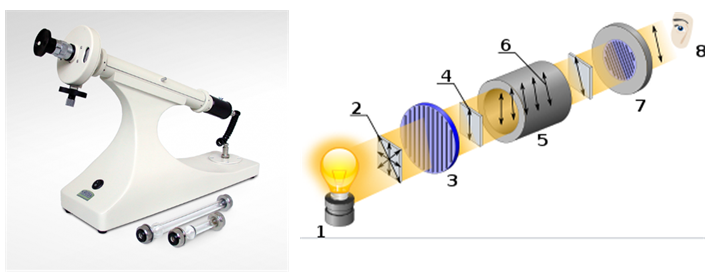Optical activity is the ability of a chiral substance to rotate the plane of polarized light. It is measured using a device called a polarimeter.Light source

 Unpolarized light

 Linear polarizer

 Linearly polarized light

 Sample cuvette

 Rotation in polarized light

 Analyzer

What is polarized light?
Normal light consists of electromagnetic waves that vibrate in all directions. When light passes through a polarizer (Nicol's prism) the electromagnetic waves vibrate in a plane. This oscillation plane coincides with the propagation plane of the wave.

Enantiomers and polarized light
When polarized light passes through a cuvette containing a chiral substance, a rotation occurs in the plane of polarization.
Chiral substances rotate polarized light and are said to be optically active (show optical activity). Those substances that do not produce rotation in polarized light are optically inactive.

right-handed and left-handed
When an optically active compound rotates in polarized light clockwise, it is said to be dextrorotatory and is represented by (+). Substances that rotate light counterclockwise are left-handed and are represented by (-). The enantiomers rotate polarized light the same angle but in opposite directions. This angle can be measured using a polarimeter.

Observed and specific optical rotation
The rotation measured in the polarimeter is called the observed optical rotation and is represented by a . Its value depends on numerous variables such as temperature, wavelength, concentration, solvent and type of substance. To avoid these dependencies the specific optical rotation [ a ] is defined.

\begin{equation}[\alpha]_{\lambda}^{t}=\frac{\alpha}{lc}\end{equation}

Where:
$\alpha$: observed optical rotation.
$[\alpha]$: specific optical rotation.
l: length of cuvette(dm).
c: concentration (g/mL).
$\lambda$: wavelength of light (line D of sodium, 589 nm) t: temperature (25ºC)

Enantiomeric excess (ee) or optical purity (PO)
When we mix two enantiomers in the same proportion, the optical rotation is zero $[\alpha] = 0$, the rotation of the dextrorotatory is compensated with that of the levorotatory (racemic mixture). If we mix enantiomers in different proportions, the optical rotation can be calculated using the enantiomeric excess or optical purity, which represents the percentage of enantiomer that causes light rotation.

\begin{equation}ee=\frac{[\alpha]_{sample}}{[\alpha]_{pure}}\cdot100\end{equation}